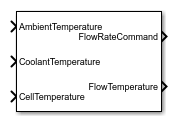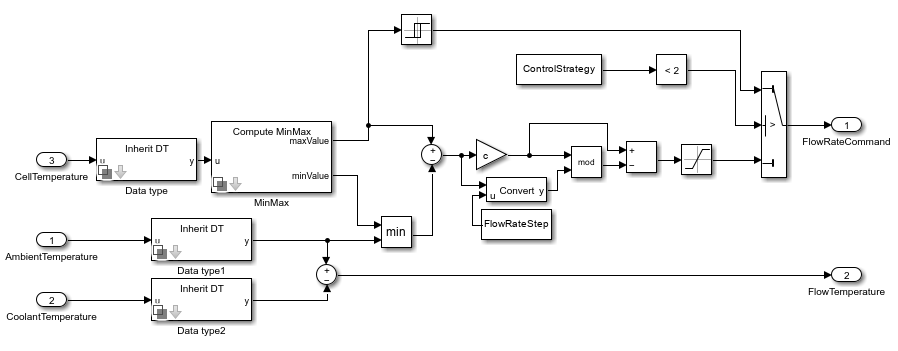# Battery Coolant Control

Battery coolant control algorithm

Since R2022b

•Libraries:
Simscape / Battery / BMS / Thermal Management

## Description

This block implements a battery coolant control algorithm.

Temperature is crucial in batteries because high temperatures significantly decrease the battery life. It is import to keep the temperature of each cell of the battery under control and activate heaters or coolers to keep the temperature within safe limits. If the temperature is too high, the battery management system (BMS) enables a cooling fan.

This block supports single-precision and double-precision floating-point simulation.

Note

To enable single-precision floating-point simulation, the data type of all inputs and parameters must be `single`.

This diagram shows the structure of the block:### Equations

The Battery Coolant Control block uses this equation to compute the flow temperature:

`$FlowTemperature={T}_{amb}-{T}_{cool}$`

where Tamb is the ambient temperature and Tcool is the coolant temperature.

If the value at the FlowRateCommand output port is equal to `0`, there is no flow in the battery. If this value is equal to `1`, then the flow rate in the battery takes its highest possible value.

If you set the Control strategy parameter to `ON-OFF control`, the block uses this equation to calculate the flow rate command:

`$FlowRateCommand=\left\{\begin{array}{cc}1,& {T}_{hottest}\ge {T}_{on}\\ FlowRateComman{d}_{old},& {T}_{off}<{T}_{hottest}<{T}_{on}\\ 0,& {T}_{hottest}<{T}_{off}\end{array}$`

where

• Thottest is the temperature of the hottest cell.

• Ton is the switch-on temperature.

• Toff is the switch-off temperature, which must be less than Ton.

• FlowRateCommandold is the flow rate command at the previous time step.

If you set the Control strategy parameter to ```Step control```, the block uses these equations to calculate the flow rate command:

`$\begin{array}{l}f\left(\Delta T\right)=c\left({T}_{hottest}-\mathrm{min}\left({T}_{coolest},{T}_{amb}-{T}_{cool}\right)\right)\\ FlowRateCommand=\mathrm{min}\left(f\left(\Delta T\right)-\mathrm{mod}\left(f\left(\Delta T\right),step\right),1\right)\end{array}$`

where

• Tcoolest is the temperature of the coolest cell.

• step is the flow rate step command. For example, if step = 0.2, the value at the FlowRateCommand output port is one of these values: [0,0.2,0.4,0.6,0.8,1].

• c controls how aggressive the coolant strategy is. This value is a constant such that, if f(ΔT)≥ 1, the value at the FlowRateCommand output port is equal to 1. Otherwise, the block scales the flow rate command linearly to `0`.

The step control strategy allows intermediate flow commands with the value you specify for the Flow rate step parameter. To compute the actual command, the block uses a function of the temperature gradient.

## Ports

### Input

expand all

Temperature of the battery cell, specified as a scalar for a single cell or a vector for multiple cells.

Temperature of the ambient, specified as a scalar.

Temperature of the coolant, specified as a scalar.

### Output

expand all

Flow rate command, returned as a scalar in the range [0, 1]. This output denotes the flow rate relative to the maximum and minimum flow rate values. A value of 0 corresponds to the minimum flow rate. A value of 1 corresponds to the maximum flow rate.

Temperature of the flow, returned as a positive or negative scalar. The total coolant inlet temperature is equal to the sum of this value and the value at the AmbientTemperature input port.

## Parameters

expand all

Control strategy for the calculation of the flow rate command.

Temperature at which the coolant pump switches on. The value of this parameter must be greater than or equal to the value of the Switch-off temperature parameter.

#### Dependencies

To enable this parameter, set Control strategy to `ON-OFF control`.

Temperature at which the coolant pump switches off.

#### Dependencies

To enable this parameter, set Control strategy to `ON-OFF control`.

Constant that controls how aggressive the coolant strategy is.

#### Dependencies

To enable this parameter, set Control strategy to `Step control`.

Step value used in the calculation of the flow rate command. The value of this parameter must be less than or equal to `1`.

#### Dependencies

To enable this parameter, set Control strategy to `Step control`.

## Version History

Introduced in R2022b